# 一. 强化学习（增强学习）的概念：

1. 监督学习(如回归，分类)

2. 非监督学习(如聚类，降维)

3. 强化学习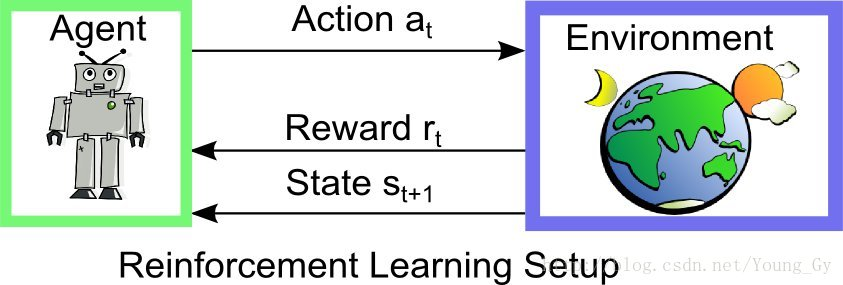# 二. Q-learning

## Q-learning思路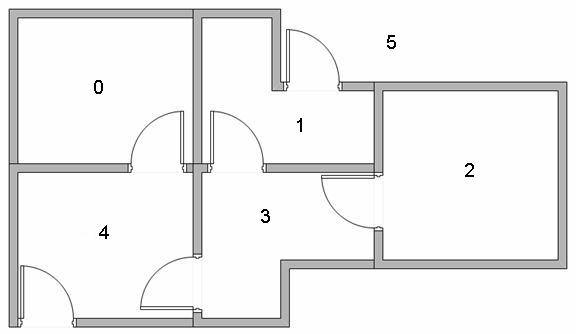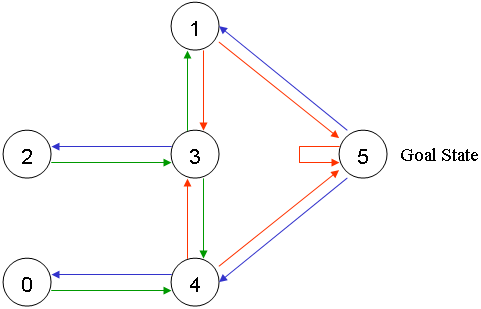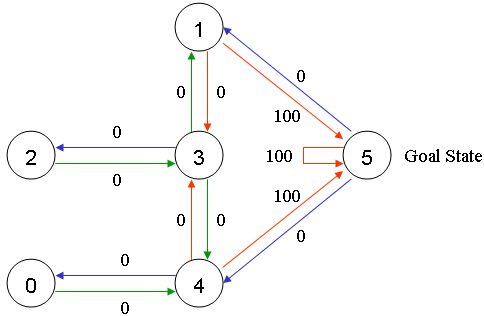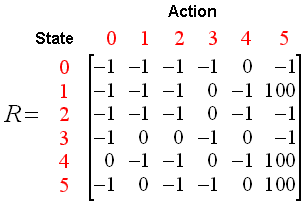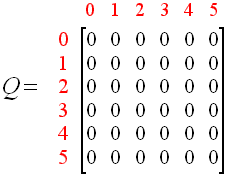Q(state, action) = Q(state, action) + α(R(state, action) + Gamma * Max[Q(next state, all actions)] - Q(state, action))

Q(state, action) = R(state, action) + Gamma * Max[Q(next state, all actions)]

• state： 表示 Agent 当前状态。
• action： 表示 Agent 在当前状态下要做的行为。
• next state： 表示Agent 在 state 状态下执行了 action 行为后达到的新的状态。
• Q(state, action)： 表示 Agent 在state 状态下执行了 action 行为后学习到的经验，也就是经验分数。
• R(state, action)： 表示 Agent 在state 状态下做 action 动作后得到的即时奖励分数。（注意是即时奖励，不是经验）
• Max[Q(next state, allactions)]： 表示 Agent 在 next state 状态下，自我的经验中，最有价值的行为的经验分数。
• Gamma：γ，表示折损率，也就是未来的经验对当前状态执行 action 的重要程度。

## Q-learning算法

Agent 通过经验去学习。Agent将会从一个状态到另一个状态这样去探索，直到它到达目标状态。我们称每一次这样的探索为一个场景（episode）。

1. 初始化 Q 矩阵，并将初始值设置成 0。

2. 设置好参数 γ 和得分矩阵 R。

3. 循环遍历场景（episode）：

（1）随机初始化一个状态 s。

（2）如果未达到目标状态，则循环执行以下几步：

1. 在当前状态 s 下，随机选择一个行为 a。

2. 执行行为 a 得到下一个状态 s。

3. 使用 Q(state, action) = R(state, action) + Gamma * Max[Q(next state, all actions)] 公式计算 Q(state, action) 。

4. 将当前状态 s 更新为 s。1.当 Agent 从 1 号房间移动到 5 号房间时，得到了奖励分数 100（即：R(1, 5) = 100）。

2.当 Agent 移动到 5 号房间后，它可以执行的动作有 3 个：移动到 1 号房间（0 分）、移动到 4 号房间（0 分）和移动到 5 号房间（0 分）。注意，这里计算的是经验分数，也就是 Q 矩阵，不是 R 矩阵！

（已经达到目标状态5，所以进入下一个场景episode）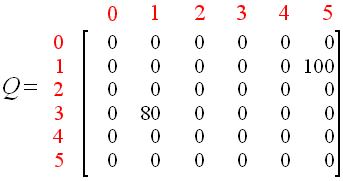## Q-learning的C语言实现：

C语言实现

#include "stdio.h"
#include "stdlib.h"
#include "time.h"

//动作数
#define ACTIONS 6
//探索次数
#define episode 1000
//目标状态，即：移动到 5 号房间。
#define target_state 5
//γ，折损率，取值是 0 到 1 之间。
#define gamma 0.8

int max(int* p, int m)
{
int i, max = *p;
for (i = 1; i < m; i++)
{
if (*(p + i) > max)
max = *(p + i);
}
return max;
}

int main()
{
// 经验矩阵。
int q = { 0 };

int r = {
{ -1, -1, -1, -1, 0, -1},
{-1, -1, -1, 0, -1, 100.0},
{ -1, -1, -1, 0, -1, -1},
{-1, 0, 0, -1, 0, -1},
{0, -1, -1, 0, -1, 100.0},
{ -1, 0, -1, -1, 0, 100.0 }
};

int start_room;
int current_state;
int current_action;
int current_action_point;
int next_state;
int step;
int next_state_max_q;

// 搜索次数。
for (int i = 0; i < episode; i++)
{
srand(time(NULL));
// Agent 的初始位置的状态。
start_room = rand() % 5;
// 当前状态。
current_state = start_room;
while (current_state != target_state)
{
//当前状态中的随机选取下一个可执行的动作。
current_action = rand() % 6;
// 执行该动作后的得分。
current_action_point = r[current_state][current_action];
if (current_action_point < 0)
{
q[current_state][current_action] = current_action_point;
}
else
{
// 得到下一个状态。
next_state = current_action;
// 获得下一个状态中，在自我经验中，也就是 Q 矩阵的最有价值的动作的经验得分。
next_state_max_q = max(&q[next_state],6);
// 当前动作的经验总得分 = 当前动作得分 + γ X 执行该动作后的下一个状态的最大的经验得分
// 即：积累经验 = 动作执行后的即时奖励 + 下一状态根据现有学习经验中最有价值的选择 X 折扣率
q[current_state][current_action] = current_action_point + gamma * next_state_max_q;
current_state = next_state;
}
}
printf("Q值表更新过程为：\n");
for (int i = 0; i < 6; i++)
{
for (int j = 0; j < 6; j++)
{
printf("%d,", q[i][j]);
}
printf("\n");
}
}
printf("\n 最终的Q值表为：\n");
for (int i = 0; i < 6; i++)
{
for (int j = 0; j < 6; j++)
{
printf("%d,", q[i][j]);
}
printf("\n");
}

start_room = rand()%5;
current_state = start_room;
step = 0;

// 这里是进行测试，依据训练好的Q值，选择动作。
while (current_state != target_state)
{
// 这里相当于python中的 np.argmax()函数，即当 np.argmax(f(x))中的f(x)取最大值的时候输出 x 的取值大小。
// 用两个for循环实现。
int m = -100;
for (int i = 0; i < 6; ++i)
{
if (m < q[current_state][i])
{//找最大值
m = q[current_state][i];
}
}
for (int j = 0; j < 6; j++)
{
if (m == q[current_state][j])
next_state = j;
}

printf("\n Agent 由 %d 号房间移动到了 %d 号房间\n", current_state, next_state);
current_state = next_state;
step += 1;
}
printf("\n Agent 在 %d 号房间开始移动了 %d 步到达了目标房间\n", start_room, step);

return 0;
}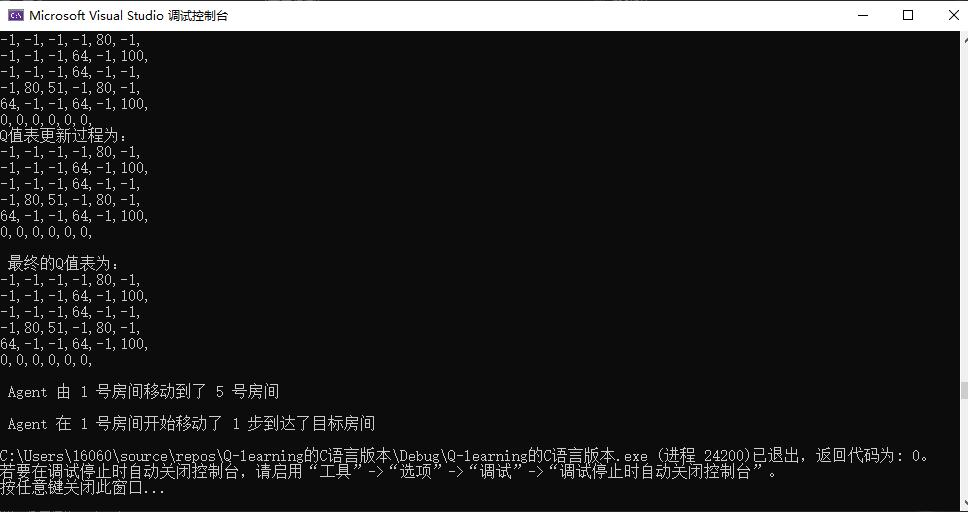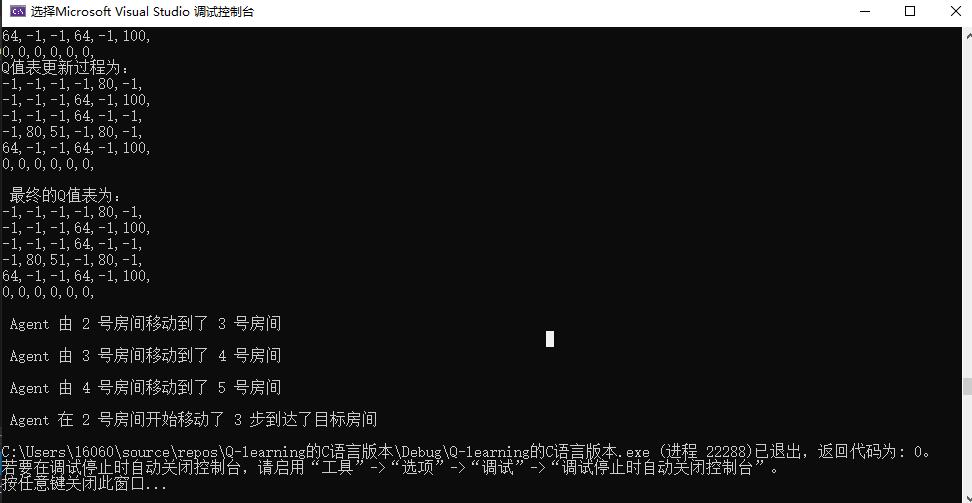GitHub链接：
https://github.com/97JOHN/Reinforcement_Learning_Train.git

 R.Sutton et al. Reinforcement learning: An introduction , 1998

 T.Mitchell. 《机器学习》，2003

 Andrew Ng.CS229: Machine learning Lecture notes

深度学习，优化与识别，2017

10-2609-15
06-199万+
03-172449
11-012452
02-251万+
02-251930